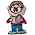## Thursday, September 27, 2007

### How Many Ways Can You Get to 100?

How many strictly positive integer solutions (x, y, z) are there, such that x + y + z = 100

1.my unofficial answer will be 5,151 combinations. Here's my thinking:

where x=0, y+z=100 | 101 combinations

where x=1, y+z=99 | 100 combinations

where x=2, y+z=98 | 99 combinations

therefore, x=n, y+z=101-n | 101-n combinations.

101
sum(x) = (101+1)(101/2)= 5151
x=0

I think that (but dont have the time to check) covers all the combinations because for each value of x, every possible value of y+z is calculated, therefore for every value of y and z, x+y and x+z = 100 respectively.

please correct me if my logic is wrong.

2.The answer is 4851 ways. It helps if you think of putting 100 stones into three piles.

By removing one stone from each pile, this is the number of ways you can arrange m-k identical stones into k (possibly empty) piles.

Now, view the k piles as a numbered set .

Write on each stone the number of a chosen pile and order the stones accordingly.

The numbered stones constitute a combination with repetition of k elements (the numbers) choose m-k (the stones). The number of combinations can be calculated as:
(m-1)!
---------
(m-k)!(k-1)!
In our case, m = 100 and k = 3, so we have 99!/97!*2! = 4851

Although, I could be wrong. ;-)

3.well technically o is not positive or negative so u can not figure one of the numbers as zero and do decimals count? because if so then the answer would b infinite

4.Hi anonymous, I'm not sure I understand your question, so forgive me. But if each of the x, y and z need to be strictly positive integers, then 0 is out and so are decimals.

5."By removing one stone from each pile, this is the number of ways you can arrange m-k identical stones into k (possibly empty) piles."

Hey there, this is a different annonymous user but when you say "possibly empty" is that erroneous considering you are leaving 0 out of the question. (Strictly positive integers)

Leave your answer or, if you want to post a question of your own, send me an e-mail. Look in the about section to find my e-mail address. If it's new, I'll post it soon.

Please don't leave spam or 'Awesome blog, come visit mine' messages. I'll delete them soon after.

Enter your Email and join hundreds of others who get their Question of the Day sent right to their mailbox

The Lamplight Manor Puzz 3-D